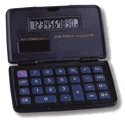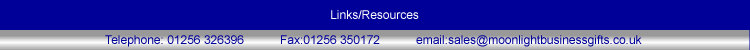# CALCULATORS02014.00 Metal Box Calculator £5.24 each (with a single colour print in one position only).02104.00 Slide Open Calculator £2.75 each (with a single colour print in one position only).02004.00 Calculator £5.33 each (with a single colour print in one position only).02107.00 Desk Calculator £1.66 each (with a single colour print in one position only).03011.00 Pocket Calculator £2.57 each (with a single colour print in one position only).04002.00 2kb Databank £5.72 each (with a single colour print in one position only).02102.50 Calculator £1.40 each (with a single colour print in one position only).02016.00 "Plutos" Pocket Calculator £4.32 each (with a single colour print in one position only).02005.00 Dual Power Calculator £1.76 each (with a single colour print in one position only).02105.50 Solar Calculator £2.12 each (with a single colour print in one position only).02007.00 Dual Power Calculator £4.11 each (with a single colour print in one position only).02009.00 Dual Power Calculator £2.23 each (with a single colour print in one position only). If you cant see the calculator you need - Just ask!